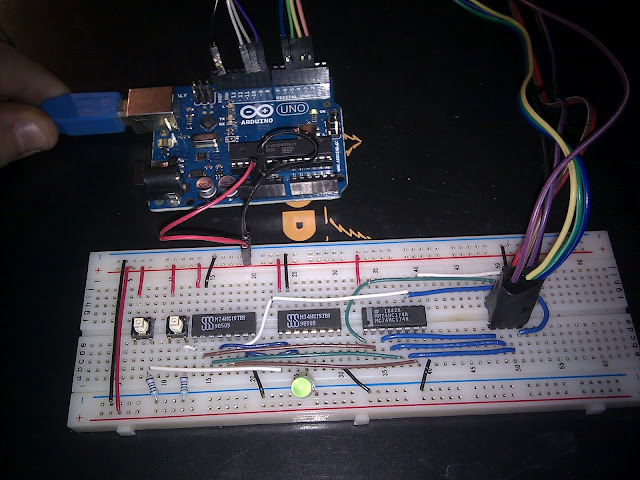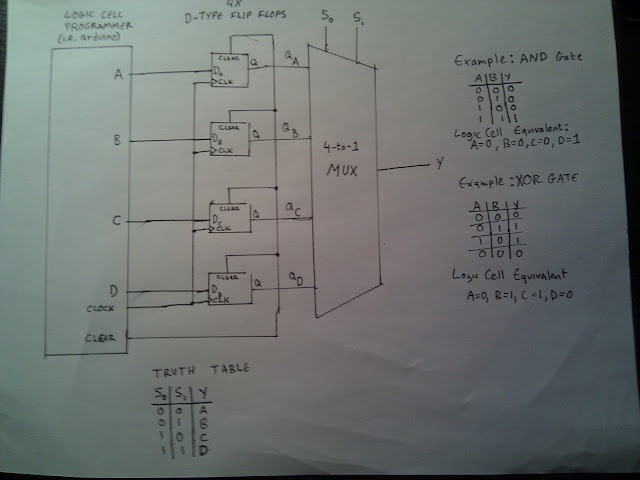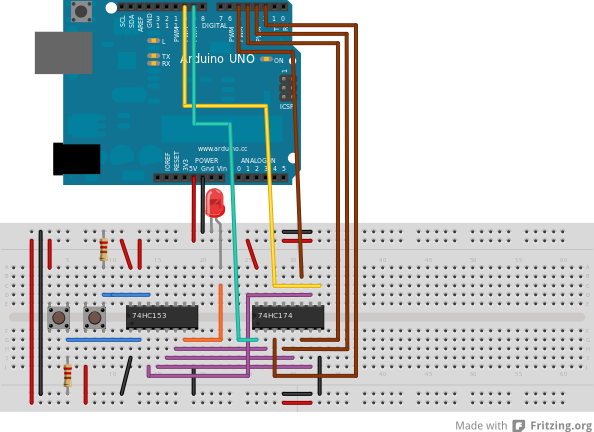## Thursday, September 20, 2012

### FPGA Logic Cells

Lately I've seen lots of homebrew CPUs mentioned in blogs and videos, but not too much about homebrew FPGAs. Did a little digging (mostly on Wikipedia), and was able to breadboard a basic logic cell circuit. Here's how I did it.A single logic cell. Using an Arduino to program the memory. Once the cell is programmed, the programming cable can be disconnected and the cell retains the truth table in memory.

## Background

A Field Programmable Gate Array is a matrix of reprogrammable logic blocks. Each logic block contains multiple logic cells.

Each cell can be programmed to function as any type of logic gate! i.e. AND, OR, XOR etc..

This matrix of blocks are then woven together in a mesh that allows blocks to interconnect with other blocks, and of course these connections can be reprogrammed any time.

Today, I will focus on the logic cell.

## Logic Cell

It performs a single boolean operation based on a pre-programmed truth table. Unlike when building gates from CMOS or TTL logic chips directly, The truth table is reprogrammable.

For 2 input truth tables, there are 2^2=4 possible outcomes. Each of those outcomes is stored in a memory. We will use four D-Type Flip Flops (74HC174) to get the memory we need.

## D-Type Flip Flop

The D-Type Flip Flop is a positive edge triggered flip flop. That means it will store the value of the data pin only when the clock pin goes high. After that, no matter what input it gets, the last value will be held, until it is cleared or a new value is clocked in. We can take 4 of these guys and tie the Clear and Clock pins together and voila - we have a 4 bit memory suitable for storing a truth table.

## Lookup Table

For example, consider an XOR gate, the four possible outcomes are (0,1,1,0)

So now that we have that in our 4-bit memory, we need a way to select one of those four values depending on what the given 2 inputs are. For that we will use a 4-to-1 Multiplexer 74HC153 (also knows as decoder or selector). Or for any of you programming nerds out there, the hardware world's equivalent to a switch statement.

The 4-to-1 multiplexer has 4 possible outcomes labeled A-D and has 2 inputs called S0 and S1, the output is labeled Y. In pseudocode it would look something like this:

If S0=0 and S1=0 then Y=A
If S0=0 and S1=1 then Y=B
If S0=1 and S1=0 then Y=C
If S0=1 and S1=1 then Y=D

Logic cells can operate both in parallel or based on a clock. So another 1-bit memory is used to store whether the cell is clocked or not.

For now I will skip the clocking part, it is easy to add it. For now I will focus on the programmable lookup table.

## SchematicExample Breadboard Layout

## Programmer Firmware

An Arduino can be used to program the 4 bit memory over a serial connection. Here is an example sketch:
```#define A      2
#define B      3
#define C      4
#define D      5

#define CLOCK 10
#define CLEAR  9
#define HOLD   8

void truth_table(bool a, bool b, bool c, bool d) {
// print truth table
Serial.println(a ? "A=0,B=0,Y=1" : "A=0,B=0,Y=0");
Serial.println(b ? "A=1,B=0,Y=1" : "A=1,B=0,Y=0");
Serial.println(c ? "A=0,B=1,Y=1" : "A=0,B=1,Y=0");
Serial.println(d ? "A=1,B=1,Y=1" : "A=1,B=1,Y=0");
Serial.println("");

// clear flip flops
digitalWrite(CLEAR, LOW);
digitalWrite(CLEAR, HIGH);

// flip bits where neccessary
digitalWrite(A, a ? HIGH : LOW);
digitalWrite(B, b ? HIGH : LOW);
digitalWrite(C, c ? HIGH : LOW);
digitalWrite(D, d ? HIGH : LOW);

// toggle clock
digitalWrite(CLOCK, HIGH);
digitalWrite(CLOCK, LOW);
}

void setup() {
pinMode(A,     OUTPUT);
pinMode(B,     OUTPUT);
pinMode(C,     OUTPUT);
pinMode(D,     OUTPUT);
pinMode(CLOCK, OUTPUT);
pinMode(CLEAR, OUTPUT);
pinMode(HOLD,  OUTPUT);

// initialize clock pin
digitalWrite(CLOCK, LOW);

// initilize clear pin
digitalWrite(CLEAR, HIGH);

// initialize truth table
truth_table(false, false, false, false);

Serial.begin(9600);
}

char values = {'F','F','F','F'};
char current;
int index = 0;

void loop() {

while (Serial.available()) {
values[index] = current;
index++;

if (index == 4) {
truth_table(values == 'T',
values == 'T',
values == 'T',
values == 'T');
index = 0;
}
}

}
```

## Using the Programmer

Open the Serial Monitor @ 9600, no line endings
To send a truth table to the device, you will need to type 4 letters, either capital "T" or capital "F".

Examples:

For an AND gate type FFFT the LED will only light up when both buttons are pressed
For a NAND gate type TTTF the LED will light up except when both buttons are pressed
For an XOR gate type FTTF the LED will only light up when only one of the buttons is pressed

Now on to logic blocks and routing :)

gap said...

- nice intro on FPGA - another thing that i'll have to get into ;-)

Jean-Charles said...

Very nice...
It's was 4 years ago, but is there another episod ?
It'll be very interesting to have blocks, then routing...
I note a difference between the first picture (3 ICs) and the schematic/fritzing breadboard..., and no use of the pin 8 (HOLD) on them...

Josh said...

@Jean Charles

I hope to add an example of blocks and routing some point soon, will comment here when I do.

Cheers!